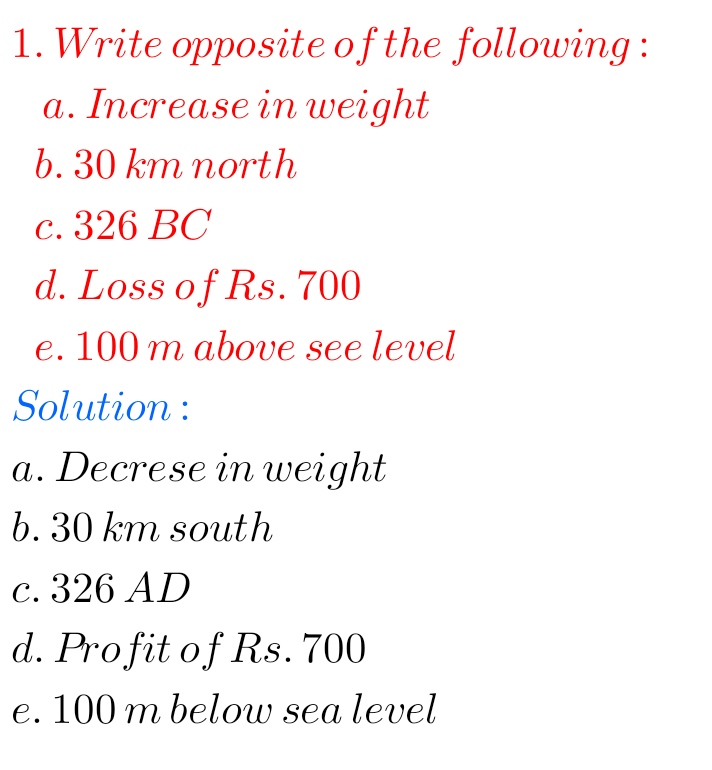# Integers

## Ncert solutions for class 7th maths chapter 1/Integers solutions,problems with solutions of Integers vii class

NCERT SOLUTIONS FOR CLASS 7th MATHS CHAPTER 1 INTEGERS Integers class 7 chapter 1 NCERT Maths solutions are given. Study the textbook lesson very well. Observe and practice example problems and solutions given in the textbook. CBSE SOLUTIONS FOR CLASS 7th MATHS CHAPTER 1 INTEGERS Important points 1. Integers are closed under addition. Integers are …## NCERT solutions for class 6th maths chapter 6/Integers/solutions for Integers, problems with solutions of Integers

NCERT SOLUTIONS FOR CLASS 6TH MATHS CHAPTER 6, INTEGERS NCERT class 6 maths chapter 6 Integers exercise 6.1 solutions are given. You should study the textbook lesson Integers very well. Observe the solutions and try them in your own method. You can also see. NCERT class 6 maths solutions Integers Exercise 6.1 Exercise 6.2 Exercise …